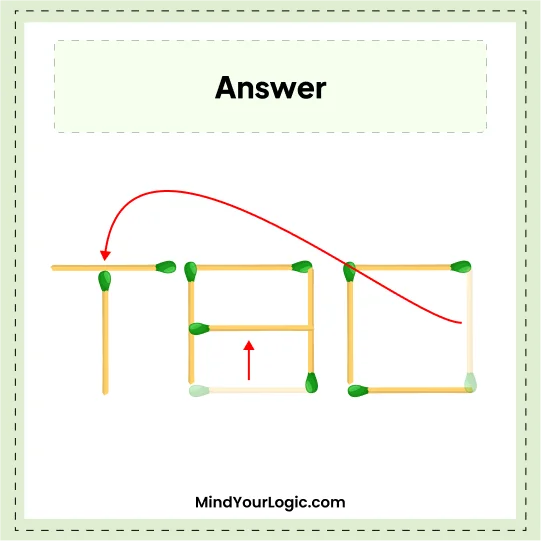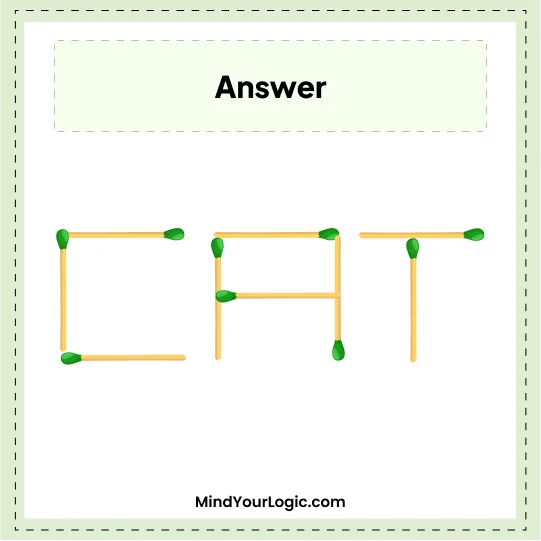We have to move one matchstick puzzles with answers, move 2 matchstick puzzles, 100+ matchstick equation puzzles, and matchstick puzzles for adults. These matchstick number puzzles will improve your spatial thinking and logical ability.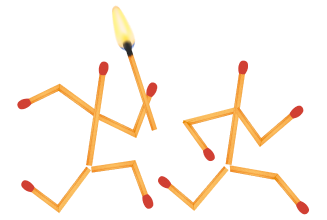#### Move 2 Matchsticks to get 11 Squares - Matchstick Puzzle

###### 46.Matchstick Puzzles
`Can you make 11 squares by just moving 2 matchsticks?`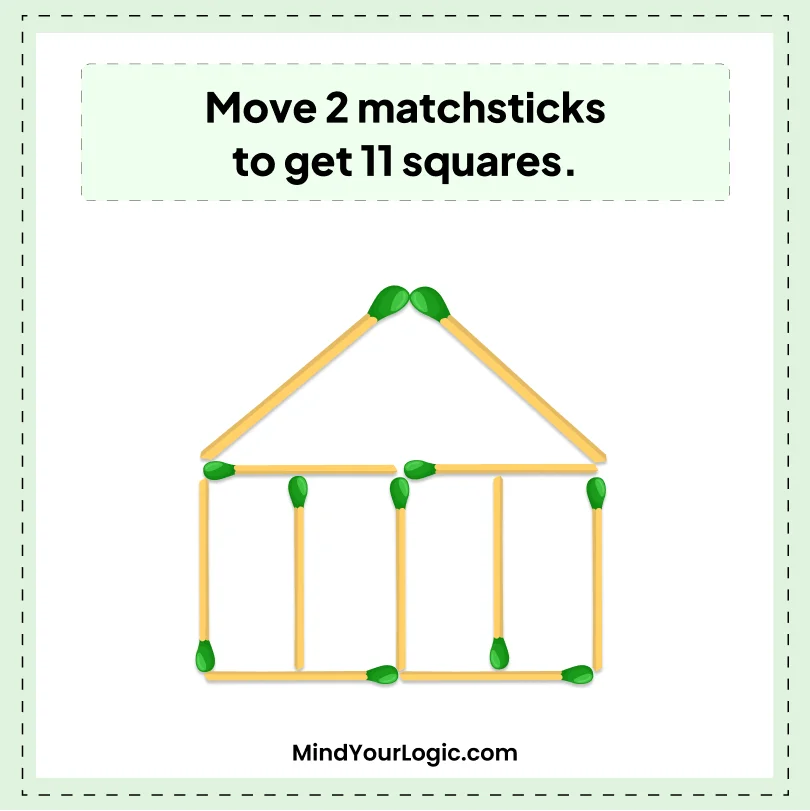•

Explanation :

``` Firstly move 2 matchsticks from roof of house and place those sticks below to get a window-like structure. So we get 11 squares.
```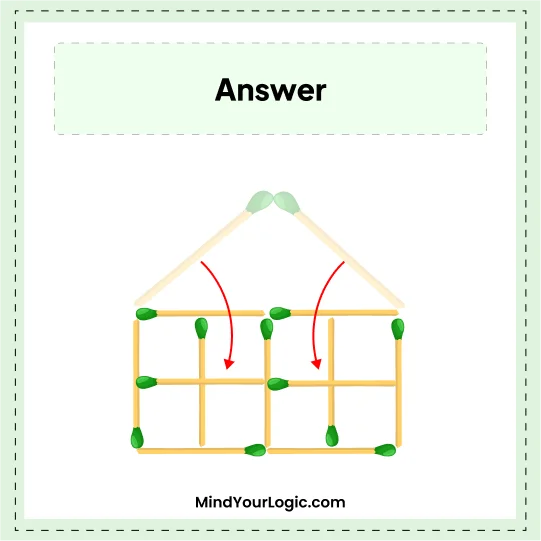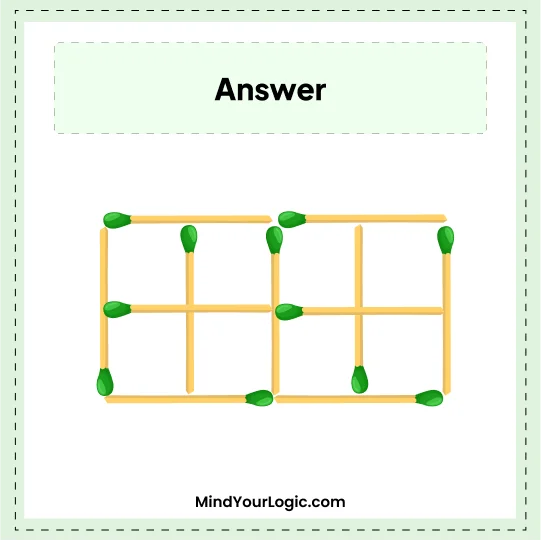#### Move 4 matchsticks to create 3 squares.

###### 47.Matchstick Puzzles
`Move 4 Matchsticks to create 3 squares.`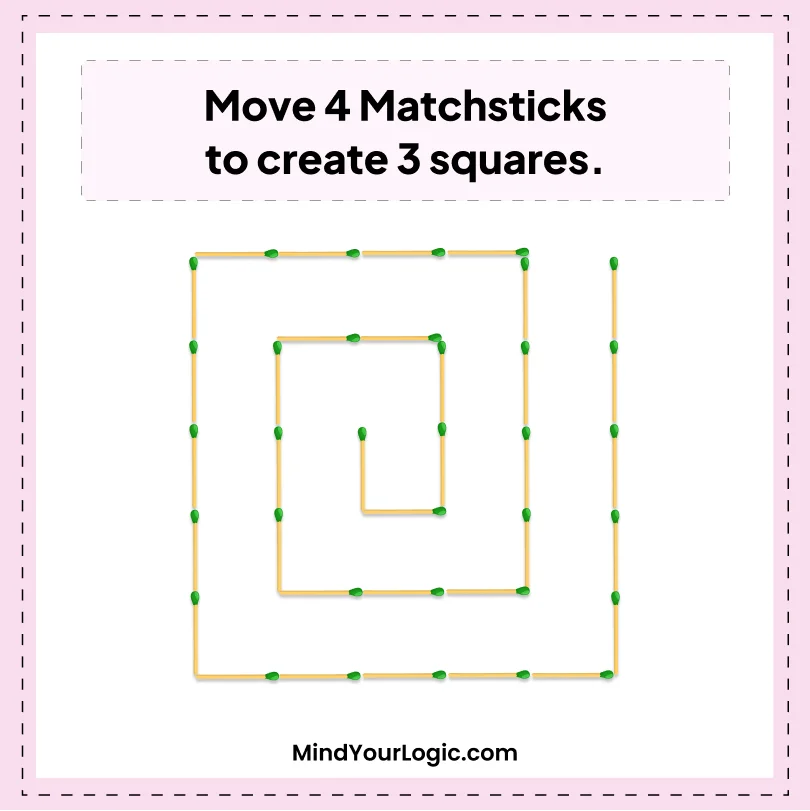•

Explanation :

``` The below image will make you understand the solution better.
We just have to shift the matchsticks shown as below images.```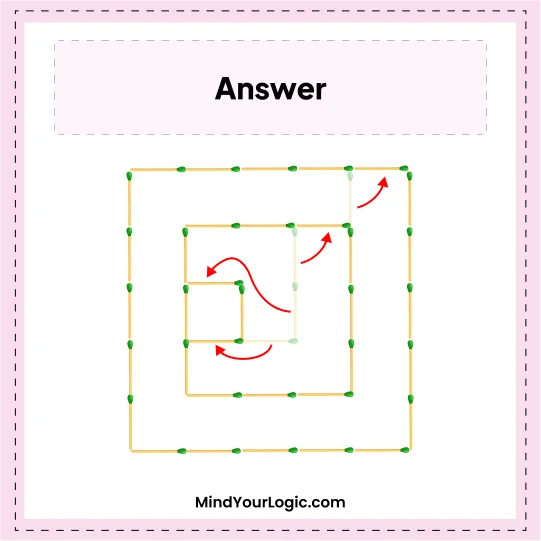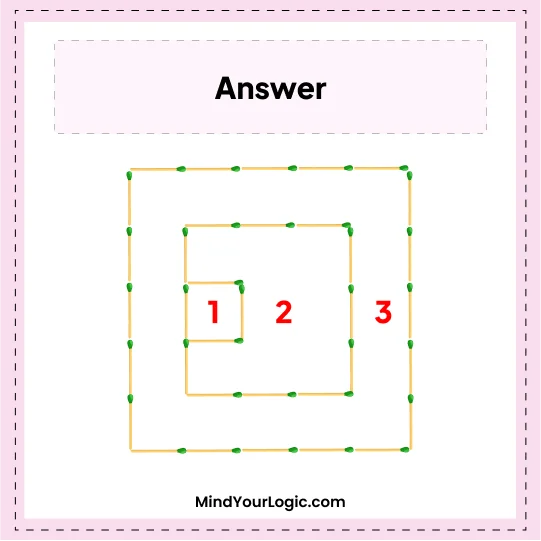#### Create another word from PAN - Matchstick Puzzle

###### 48.Matchstick Puzzles
`Remove one matchstick to create another word.`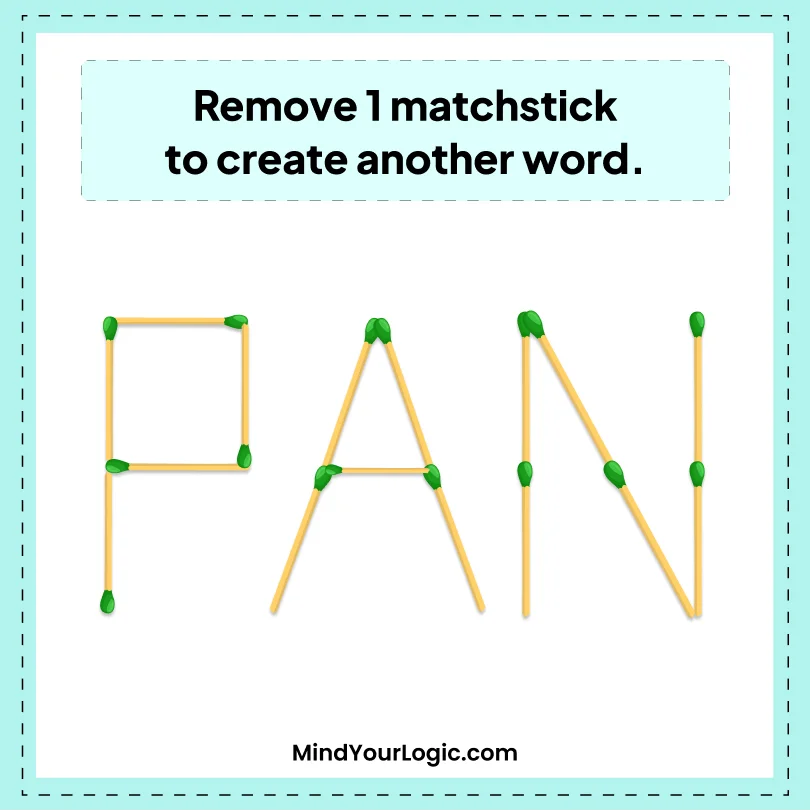•

Explanation :

``` The solution to this puzzle is very simple, the question says we have to remove one stick to create another word.
So, the question says pan, just remove one stick from "P" which becomes "F" and we get another word FAN.```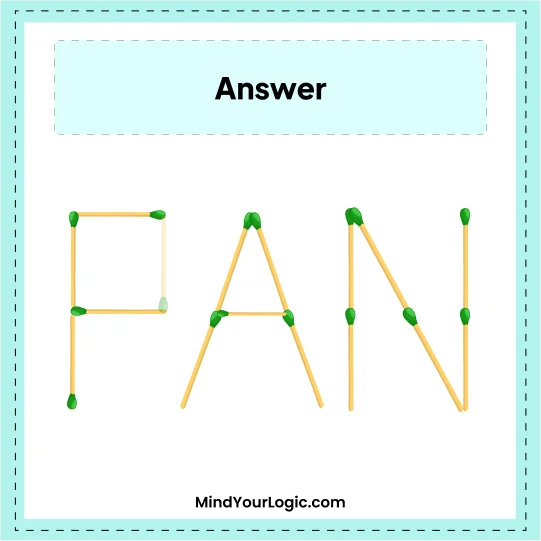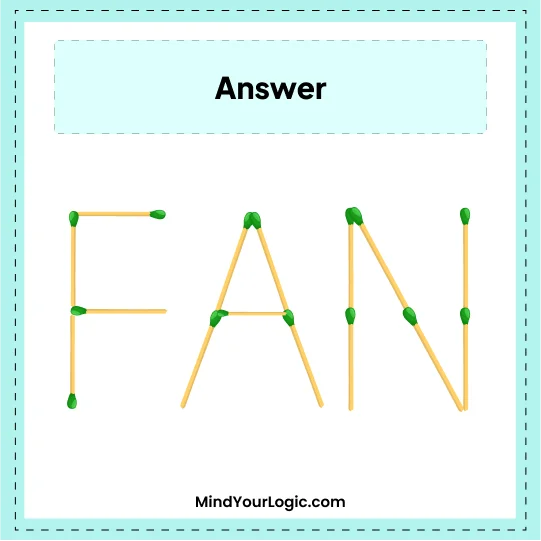#### Move 3 matchsticks and make 2 squares - Matchstick Puzzle

###### 49.Matchstick Puzzles
`Try to solve this puzzle just by moving only 3 matchsticks to make 2 squares. `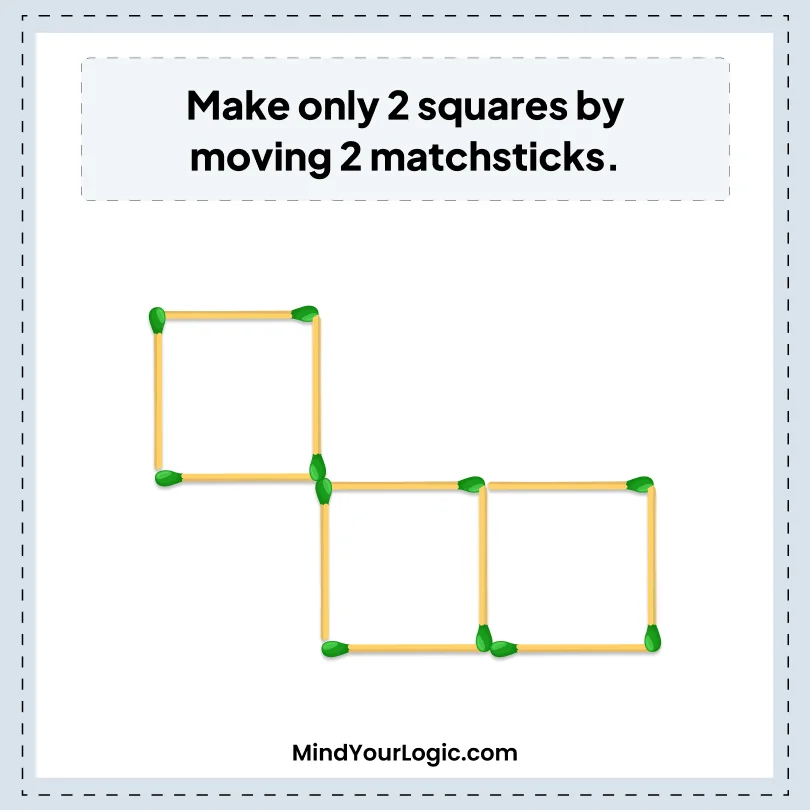•

Explanation :

` The Bottom 2 square sticks have to be shifted above with 1st square at the top and the middle stick has to be placed at the right top.`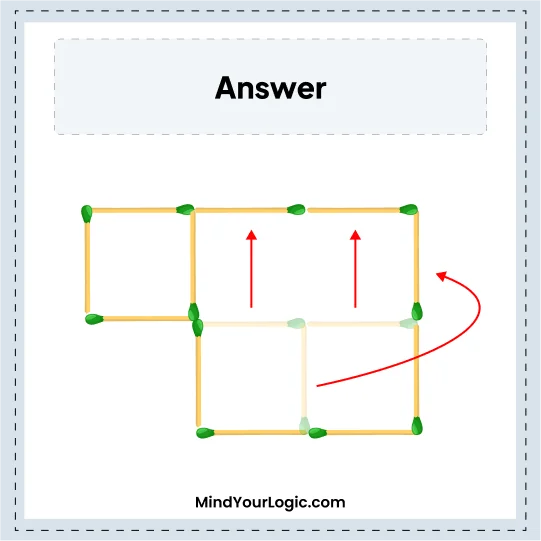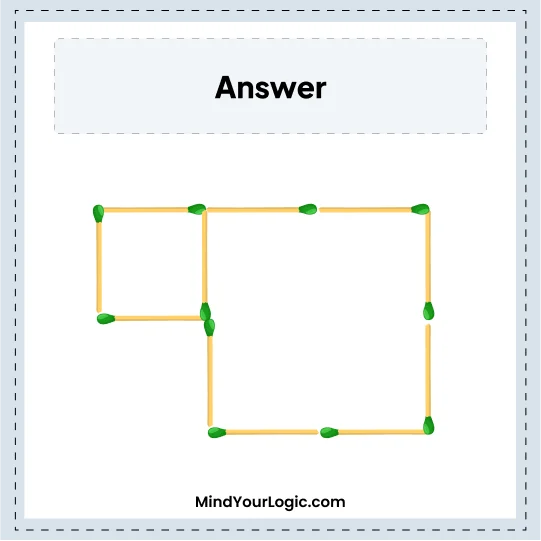#### Move 2 to Create Another word from 100 - Matchstick Puzzle

###### 50.Matchstick Puzzles
`Move only 2 matchsticks, change 100 into a word CAT.`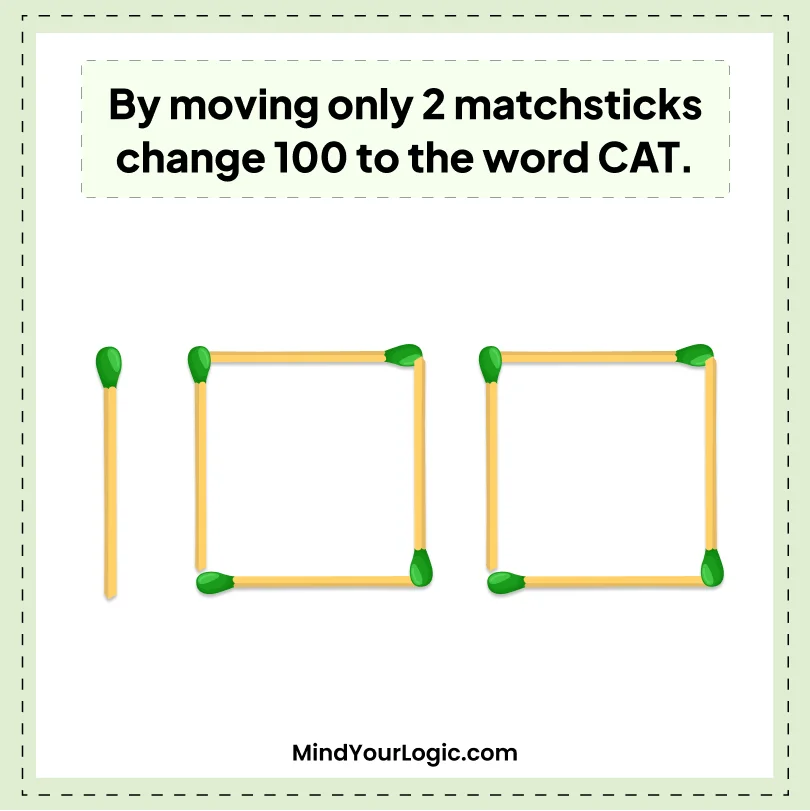•

Explanation :

``` Let"s solve the puzzle,
1. Remove 1 stick from the last zero (0) that becomes "C" shift that sticks to the top of number 1 which becomes "T".
2. From middle zero shift the stick to the middle, which becomes "A" Word "CAT". We get in "TAC" format and rearrange them in the correct order. ```